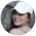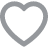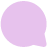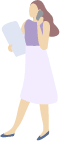4 Ways to Use a Decision Making Matrix (Plus Examples) | FairygodbossMystery Woman
Tell us more for better jobs, advice
and connections
Discover and join groups with like-minded women who share your interests, profession, and lifestyle.
COMPANIES YOU FOLLOW
Get alerted when there are new employee reviews.
Get notified when new jobs are posted.
Smart Tactics
4 Ways a Decision-Making Matrix Can Optimize Your Career ChoicesAnnaMarie Houlis,3.66k
Journalist & travel blogger71 Comment

Making decisions is no easy feat. In fact, many of us struggle with decision anxiety and decidophobia, which is the fear of making decisions.

That's where a decision-making matrix can come in handy. Here's what you should know about what a decision-making matrix is, as well as why, when and how to use one — with examples for your reference!

## What is a decision-making matrix?

A decision-making matrix is, in short, a useful technique to help you make a decision — particularly when you're deciding between a number of solid alternatives and have a variety of different factors to take into account.

Simply, a decision-making matrix, also known as a decision matrix analysis, works like this: You list your options as rows on a table, and you list the factors you'll need to consider for each as columns. Then, you score each option/factor combination — and weight this score by the relative importance of the factor. Then you add up all of the scores to give you an overall score for each option that should, in theory, help you to make a more informed decision.

## Why should you use a decision-making matrix?

You should use a decision-making matrix because it can really help you make a more informed decision that ensures that you have taken into account all necessary factors and have exhausted all of your options. A decision-making matrix will ease some of the stress of making an otherwise tough decision by clearing spelling out your best options based on a final weighted score.

## When should you use a decision-making matrix?

You can use a decision-making matrix when you're trying to make any decision at all. That said, it's most useful, again, when you have a multitude of good options from which to choose and/or a lot of different factors to consider.

For example, you might use a decision-making matrix when you're buying a car and have to factor in cost, perhaps mileage already on the car (or mileage you plan to put on it), fuel type and cost, the cost of insurance for the car, the sound system, the navigation, the interior material, the color, the size, etc.

You might also use a decision-making matrix when you're buying a new home and you have to factor in cost (of both the down payment and your mortgage), location, size, necessary repair costs, furnishings, proximity to family/work/schools/parks/etc., income taxes, the yard size (or lack thereof), the garage size (or lack thereof), the number of floors, the number of bathrooms, the number of bedrooms, etc.

You might also use a decision-making matrix when you're shopping for a wedding venue and you have to factor in cost, what's included (and what's not included), location, availability (or lack thereof) for your preferred dates, size, guest capacity, aesthetics, etc.

## How can you make a decision-making matrix?

A decision-making matrix is easy to make. Follow these five simple steps.

### 1. List your options and factors.

List all of your options rows in a table. Then list all of the factors that you need to consider as the column heads.

### 2. Score each option.

Work your way down the columns of your table and give each option a score for the different factors, from 0 (very poor) to 5 (very good). For example, if you're buying a car and the cost is a factor, perhaps you score the Mercedes a 1 because it's quite pricy, but you score the Toyota a 4 because it's on the more affordable end of the scale.

### 3. Value the importance of the factors.

Determine the relative importance of each factor in your decision, from 0 (not at all important) to 5 (really important). For example, perhaps the cost of your car isn't that important to you, though it is a determining factor still — you might give it a 2.

### 4. Multiply your option scores by the weight of the factors' importance.

Simply multiply each of your scores for each option (step 2) by the values that you placed on each factor (step 3). The result will be a weighted score for each option/factor combination.

## 5. Add up the weighted scores for each option.

Once you've completed step 4 for all of your options, add up all of the weighted scores for each of your options. The option with the biggest final weighted score will be your best option.

## What are some examples of decision-making matrixes?

Here are some examples of decision-making matrixes to get you started.

### 1. Work

Here's an example of a decision-making matrix for a working professional looking to change careers.

 Salary (5) Work-Life Balance (4) Benefits (3) Title (5) Location (3) Job Offer A 5 1 5 4 1 Job Offer B 2 4 4 1 3 Job Offer C 3 3 4 3 5

Scores:

• Job Offer A: 5 x 5 = 25; 4 x 1 = 4; 3 x 5 =15; 5 x 4 = 20; 3 x 1 = 3 | 25 + 4 + 15 + 20 +3 = 67
• Job Offer B: 5 x 2 = 10; 4 x 4 = 16; 3 x 4 = 12; 5 x 1 = 5; 3 x 3 = 9 | 10 + 16 + 12 + 5 + 9 = 52
• Job Offer C: 5 x 3 = 15; 4 x 3 = 12; 3 x 4 = 12; 5 x 3 = 15; 3 x 5 = 15 | 15 +12 +12 + 15 + 15 = 69

In this case, Job Offer C wins. Even though it doesn't score so well in salary or title, it averages the best scores based on the weighted value of each factor.

### 2. Student

Here's an example of a decision-making matrix for a student choosing a college.

 Location (3) Majors (5) Cost (4) Size (2) Prestige (4) Career Center (3) College A 5 3 3 4 5 2 College B 3 3 5 2 3 1 College C 4 2 5 3 4 5

Scores:

• College A: 3 x 5 = 15; 5 x 3 = 15; 4 x 3 = 12; 2 x 4 = 8; 4 x 5 =20; 3 x 2 = 6 | 15 + 15 + 12 + 8 + 20 +6 = 76
• College B: 3 x 3 = 9; 5 x 3 = 15; 4 x 5 = 20;  2 x 2 = 4; 4 x 3 = 12; 3 x 1 = 3 | 9 + 15 + 20 + 4 + 12 + 3 = 63
• College C: 3 x 4 = 12; 5 x 2 = 10; 4 x 5 = 20; 2 x 3 = 6; 4 x 4 =16; 3 x 5 = 15 |12 + 10 + 20 + 6 + 16 + 15 = 79

In this case, College C wins. Even though it doesn't score so well in major options or size, it averages the best scores based on the weighted value of each factor.

### 3. Personal

Here's an example of a decision-making matrix for someone looking to rent an apartment.

 Location (5) Size (4) Rent (4) Broker's Fee (2) Amenities (4) Apartment A 5 1 1 3 4 Apartment B 5 2 3 2 5 Apartment C 4 3 3 1 4

Scores:

• Apartment A: 5 x 5 = 25; 4 x 1 = 4; 4 x 1 = 4; 2 x 3 = 6; 4 x 4 = 16 | 25 + 4 + 4 + 6 +16 = 55
• Apartment B: 5 x 5 = 25; 4x 2 = 8; 4 x 3 = 12; 2 x 2 = 4; 4 x 5 = 20 | 25 + 8 +12 + 4 +20 = 69
• Apartment C: 5 x 4 = 20; 4 x 3 = 12; 4 x 3 = 12; 2 x 1 = 2; 4 x 4 = 16 | 20 + 12 + 12 + 2 + 16 = 62

In this case, Apartment B wins. Even though it doesn't score so well in size or for the broker's fee, it averages the best scores based on the weighted value of each factor.

--

AnnaMarie Houlis is a feminist, a freelance journalist and an adventure aficionado with an affinity for impulsive solo travel. She spends her days writing about women’s empowerment from around the world. You can follow her work on her blog, HerReport.org, and follow her journeys on Instagram @her_report, Twitter @herreportand Facebook.71 Comment
1 CommentThe Fairygodboss Feed